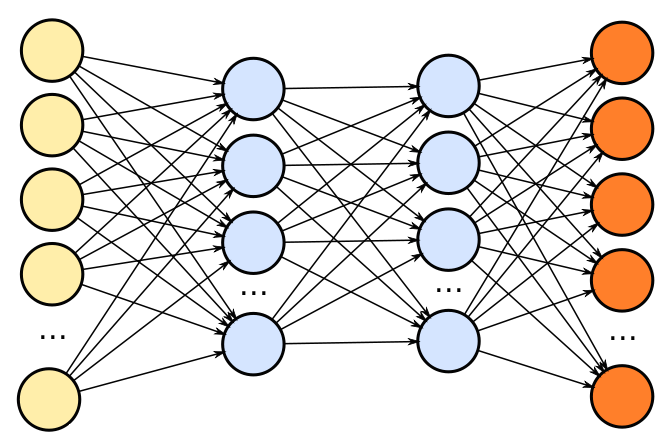# How to train Neural NetworksIn this post, I am going to write about the general blueprint to be followed for any deep learning model. Here I am not going in-depth into deep learning concepts but this acts as a basic step that can be followed to develop neural networks.

In this post, I am going to write about the general blueprint to be followed for any deep learning model. Here I am not going in-depth into deep learning concepts but this acts as a basic step that can be followed to develop neural networks. Some steps may be added or can be removed from the below list based on the requirement.

1 . Data preprocessing

The data we get for modeling is most of the time unstructured and raw, where we have lots of data that is not required for our case. So we need to keep the data which is necessary and leave out the rest

2. Weight initialization

The first step comes in modeling a neural network is weight initialization and this is an extremely important step because if the weights are not initialized properly then converging to minima is impossible, but if done is the right way then optimization is achieved in the least time. There are several techniques such as zero initialization, random initialization, HE initialization, glorant initialization, Xavier, etc.

3. Choose the right activation function

The activation function is considered as a gate that can be as simple as on or off or it can transform the input of the neuron and give output. There are several types of activation functions that you can choose based on the use cases. Some of the activation functions are broadly categorized into Linear and nonlinear. The problem with linear activation functions is that backpropagation cannot be applied. And if there are multiple layers of a linear transformation, it is still equivalent to one layer because the function of linear is still a linear function. We have non-linear activations like, Sigmoid, tanh, ReLu which solves the problems of linear activation functions.

## Deep Learning 101 —  Neural Networks Explained

The past few decades have witnessed a massive boom in the penetration as well as the power of computation, and amidst this information.

## Explain Deep Learning Neural Networks to your grandma

“You do not really understand something unless you can explain it to your grandmother” Not sure where this quote originally came from, it is sometimes kind of half-attributed to Albert Einstein.

## Deep Learning Explained in Layman's Terms

Deep Learning Explained in Layman's Terms. In this post, you will get to learn deep learning through a simple explanation (layman terms) and examples.

## Alibaba’s Mobile Neural Network: A deep learning framework for mobile and embedded devices

A peek at Alibaba’s Mobile Neural Network (MNN) and how it achieves balance between high performance, flexibility, and ease-of-use.

## Artificial Neural Networks — Recurrent Neural Networks

Artificial Neural Networks — Recurrent Neural Networks. Remembering the history and predicting the future with neural networks. A intuition behind Recurrent neural networks.